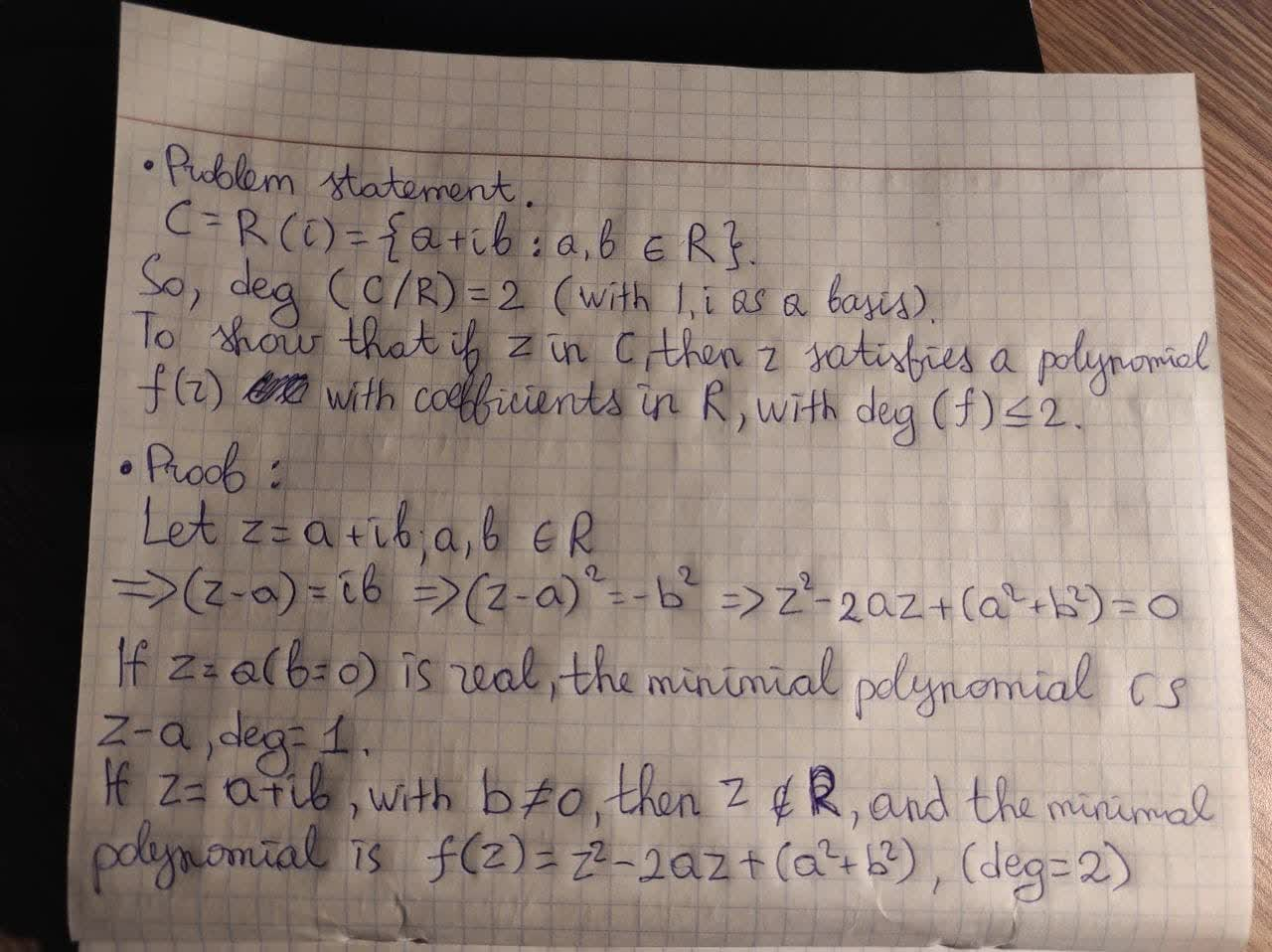Show that if K sube F is an extension of degree k, every element alpha in F has a minimal polynomial over K of degree <=k. Find theminimal polymials of complex numers over RR explicitly to verify this factJosalynn 2020-11-09 Answered

Show that if $$\displaystyle{K}\subseteq{F}$$ is an extension of degree k, every element $$\displaystyle\alpha\in{F}$$ has a minimal polynomial over K of degree <=k. Find theminimal polymials of complex numers over $$\displaystyle\mathbb{R}$$ explicitly to verify this fact

• Questions are typically answered in as fast as 30 minutes

Solve your problem for the price of one coffee

• Math expert for every subject
• Pay only if we can solve itnitruraviX
To show that the degree of the minimal polynomial of any complex number over R is of degree at most 2.
This is the problem statement.
Now, $$\displaystyle\mathbb{C}=\mathbb{R}{\left({i}\right)}={\left\lbrace{a}+{i}{b}:{a},{b}\in\mathbb{R}\right\rbrace}.$$
So, $$\displaystyle{d}{e}{g{{\left(\frac{\mathbb{C}}{\mathbb{R}}\right)}}}={2}$$, (with I, i as a basis).
To show that if $$\displaystyle{z}\in\mathbb{C}$$, then z satisfies a polynomial f(z) with coefficients in $$\displaystyle\mathbb{R}$$ with deg(f)
Proof of the statement. To start with any z satisfies a polynomial equation of degree less than or equal to 2.
Let $$\displaystyle{z}={a}+{i}{b},{a},{b}\in\mathbb{R}$$
$$\displaystyle\Rightarrow{\left({z}-{a}\right)}-{i}{b}\Rightarrow{\left({z}-{a}\right)}^{{2}}=-{b}^{{2}}\Rightarrow{z}^{{2}}-{2}{a}{z}+{\left({a}^{{2}}+{b}^{{2}}\right)}={0}$$
Completing the proof
If z=a (b=0) is real, the minimal polynomial is z-a, deg=1
If z=a+ib, with $$\displaystyle{b}\ne{0}$$, then $$\displaystyle{z}\notin\mathbb{R}$$, and the minimal polynomial is $$\displaystyle{f{{\left({z}\right)}}}={z}^{{2}}-{2}{a}{z}+{\left({a}^{{2}}+{b}^{{2}}\right)}$$, (deg=2).